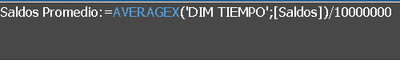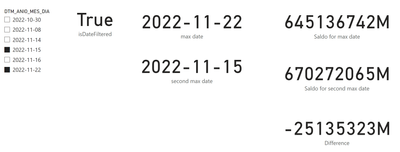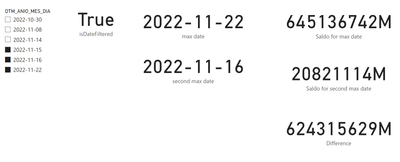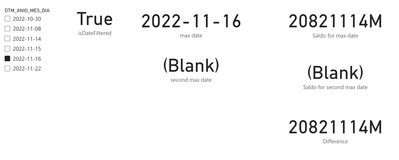cancel
Showing results for
Did you mean:Regular Visitor

## calculate Delta dynamic different dates

Hello!

I have a problem with a delta calculation and I have not been able to give a solution, I hope someone can help me and thanks in advance!

What do I require:
I require a calculation for the variation of the current month vs the previous month
and one for the variation of the current day vs the previous day
All this regarding a measure called [Saldos Promedio]

Issues:
1. If there are no filters, the measurements must calculate the variation of the current day vs. the previous day or the current month vs. the previous month
but if 2 different months or days (not consecutive) are filtered, you must calculate the variation.
2. The dates are not consecutive in my master date table for example (current day 2023-01-10 previous day: 2023-01-05) this is because the data loaded is only for business days.

I attach screenshots of the date table.the measure [Saldos Promedio] is a SumX of the balances column for each date of the Dim Tiempo table1 ACCEPTED SOLUTIONSolution Sage
Due to fact that your sample data contains a rows only for 2 months (Oct and Nov) I will show you how to do that on dates and you can simply do that same for months 🙂

Helper measuers:
This measure is not needed for that case, but maybe you will need that later to check if you you want difference for dates or months (depending what is filtered).

max date = lastdate(SampleData[DTM_ANIO_MES_DIA])

second max date =
var max_date = [max date]
return CALCULATE([max date],FILTER(ALLSELECTED(SampleData),SampleData[DTM_ANIO_MES_DIA]<max_date))

Saldo = SUM(SampleData[saldo diario])​

Measures:

``````Saldo for max date =
var ref_date = [max date]
return CALCULATE([Saldo],SampleData[DTM_ANIO_MES_DIA]=ref_date)``````
``````Saldo for second max date =
var ref_date = [second max date]
return CALCULATE([Saldo],SampleData[DTM_ANIO_MES_DIA]=ref_date)``````
``Difference = [Saldo for max date] - [Saldo for second max date]``

Example #1:

Non of dates selected:Example #2:

Two dates are selected.Example #3:

Three dates are selected (note that it only shows value for 2 latest one)Example #4:

One date selected (nothing to compare to). What to do?4 REPLIES 4Solution Sage

Hi,

can you use https://wetransfer.com/ to provide some sample data (image is not useful, because we need to recreate some sample data)?

What I understood:

you need ONE measure that (based on the user selection) switch calculation betweeen delta month and previous month (or 2nd month) and delta day and 2nd max day?

Yes, it can be done, but it will be easier on sample data. 🙂Regular Visitor

sure!

this is the link of the sample data:

not necessarily a single measure

It can be one for the variation between months
and one for the variation between days

for example

filter October and December and that the measurement shows the difference between these
but if there is no filter that shows me the difference between December and November

same case for the days
If there is a filter of two dates, then show me the difference, for example, the balance of 10/30/22 vs 11/11/2022
If there are no filters, show me the last 2 dates that exist in the table

Thank you!Solution Sage
Due to fact that your sample data contains a rows only for 2 months (Oct and Nov) I will show you how to do that on dates and you can simply do that same for months 🙂

Helper measuers:
This measure is not needed for that case, but maybe you will need that later to check if you you want difference for dates or months (depending what is filtered).

max date = lastdate(SampleData[DTM_ANIO_MES_DIA])

second max date =
var max_date = [max date]
return CALCULATE([max date],FILTER(ALLSELECTED(SampleData),SampleData[DTM_ANIO_MES_DIA]<max_date))

Saldo = SUM(SampleData[saldo diario])​

Measures:

``````Saldo for max date =
var ref_date = [max date]
return CALCULATE([Saldo],SampleData[DTM_ANIO_MES_DIA]=ref_date)``````
``````Saldo for second max date =
var ref_date = [second max date]
return CALCULATE([Saldo],SampleData[DTM_ANIO_MES_DIA]=ref_date)``````
``Difference = [Saldo for max date] - [Saldo for second max date]``

Example #1:

Non of dates selected:Example #2:

Two dates are selected.Example #3:

Three dates are selected (note that it only shows value for 2 latest one)Example #4:

One date selected (nothing to compare to). What to do?Regular Visitor

Thank you!

It works perfectly, I replicated the measurements to calculate the months and it worked perfectly!